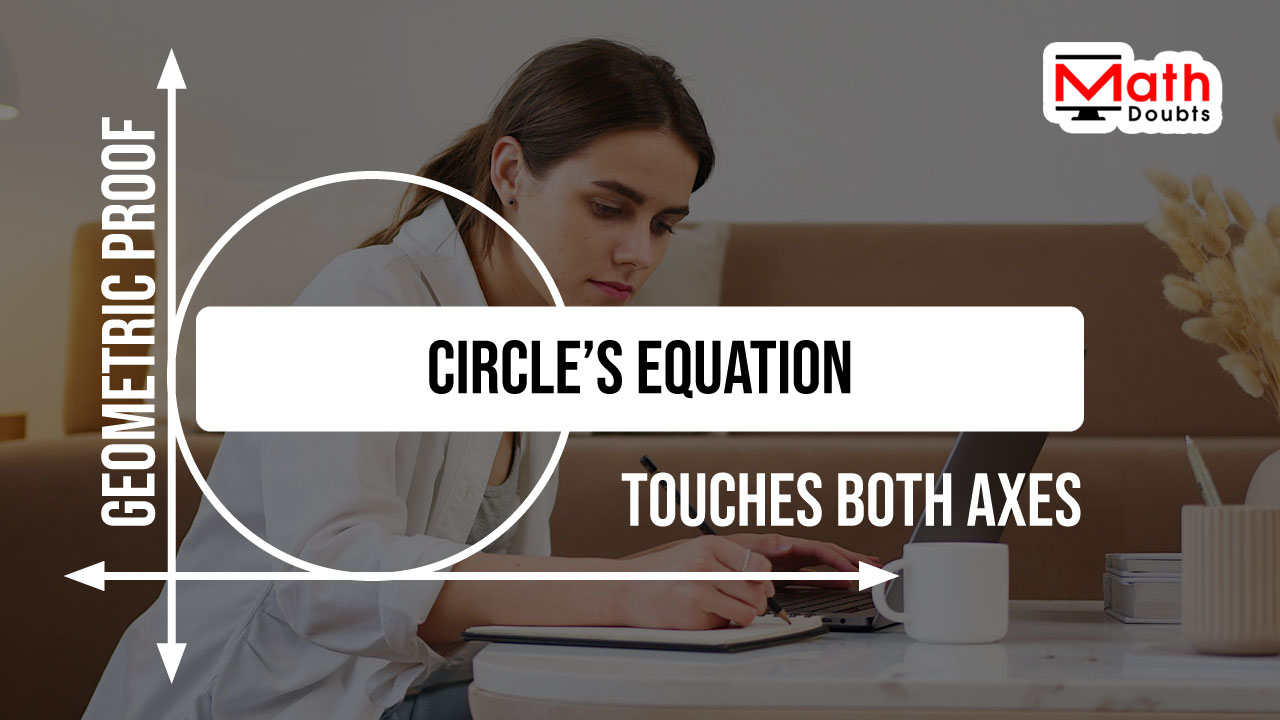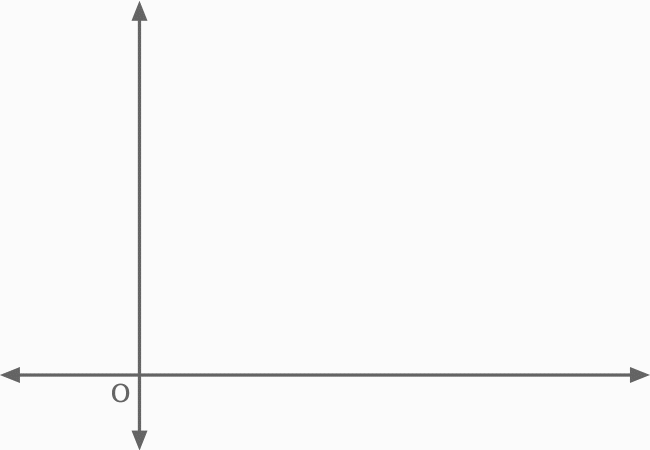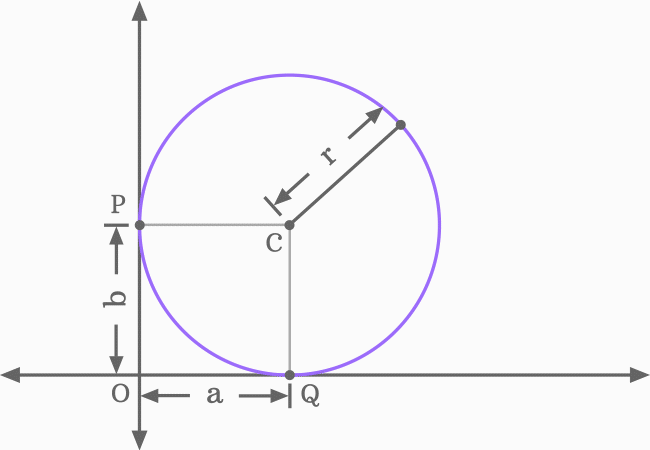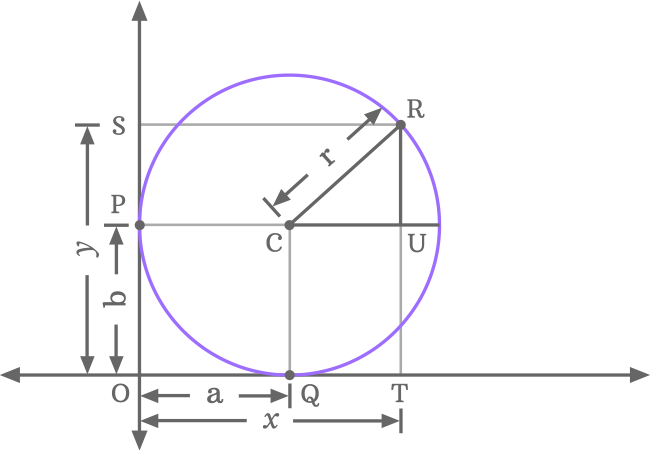# Proof of Equation of a circle touches both axesThe equation of a circle touches both axes, is written in the following three forms in mathematics.

$(1).\,\,$ $(x-a)^2+(y-a)^2$ $\,=\,$ $a^2$

$(2).\,\,$ $(x-b)^2+(y-b)^2$ $\,=\,$ $b^2$

$(3).\,\,$ $(x-r)^2+(y-r)^2$ $\,=\,$ $r^2$

You can use represent the circle touching the both axes by any one of the above three equations. So, let us learn how prove the equation of a circle touches both axes of two dimensional cartesian coordinate system in mathematical form geometrically.

### Construction of a Triangle inside a Circle

A right triangle must be constructed inside a circle when the circle is touching both $x$-axis and $y$-axis in a quadrant of the two dimensional cartesian coordinate system and it is useful to derive the mathematical equation of a circle, which touches both axes.There are five geometrical steps to construct a right angled triangle inside a circle when the circle touches both $x$-axis and $y$-axis of the two dimensional space.

1. Draw a circle in such away that it touches the $x$-axis at point $P$ and also touches the $y$-axis at point $Q$ in a two dimensional space.
2. Denote the center or centre of a circle by $C$. Let’s assume that the $x$ and $y$ coordinates of the center are $a$ and $b$ respectively. Hence, the centre is written as $C(a, b)$ in coordinate form in mathematics.
3. Consider a point on the circle and it is denoted by $R$. The coordinates of the point $R$ are denoted by $x$ and $y$ respectively. Therefore, the point $R$ is mathematically written as $R(x, y)$ in coordinate form.
4. Connect the center $C$ and point $R$ by drawing a straight line and the line segment $\overline{CR}$ represents the radius of the circle. Let’s assume that the radius of the circle is $r$ units.
5. Now, draw a horizontal line from the center to intersect the circle at a point. Similarly, draw a vertical line from point $R$ to intersect the horizontal line perpendicularly at a point $U$. It forms a right angled triangle, written as $\Delta UCR$ geometrically.

### Relation of Center’s coordinates with Radius

It is time to understand the geometrical relationship between the radius and the coordinates of the circle.The distance from center to point $Q$ is the radius of the circle and it is exactly equal to the distance from origin to point $P$. Actually, it is the $y$-coordinate of the centre of the circle.

$\implies$ $CQ \,=\, OP$

$\,\,\,\therefore\,\,\,\,\,\,$ $r \,=\, b$

Similarly, the distance from point $P$ to centre is the radius of the circle and it is exactly equal to the distance from the origin to the point $Q$. In fact, it is the $x$-coordinate of the center of the circle.

$\implies$ $OQ \,=\, PC$

$\,\,\,\therefore\,\,\,\,\,\,$ $a \,=\, r$

The above two mathematical equations are cleared that the $x$-coordinate and $y$-coordinate are exactly equal to the radius of the circle.

$\,\,\,\therefore\,\,\,\,\,\,$ $a$ $\,=\,$ $b$ $\,=\,$ $r$

### Measure the Lengths of sides of Right triangle

In $\Delta UCR$, the opposite side is $\overline{RU}$, the adjacent side is $\overline{CU}$ and the hypotenuse is $\overline{CR}$, and their lengths are written as $RU, CU$ and $CR$ respectively.Now, let us find the length of every side of the right angled triangle mathematically.

1. $CU$ $\,=\,$ $QT$ $\,=\,$ $OT\,–\,OQ$ $\,=\,$ $x\,–\,a$
2. $RU$ $\,=\,$ $SP$ $\,=\,$ $OS\,–\,OP$ $\,=\,$ $y-b$
3. $CR \,=\, r$

### Express the Relationship between the sides

According to the Pythagorean Theorem, the lengths of all three sides of right triangle $UCR$ can be written mathematically in an equation.

${CR}^2$ $\,=\,$ ${CU}^2+{RU}^2$In this equation, $CU, CR$ and $RU$ represent the lengths of adjacent side, hypotenuse and opposite side. Now, substitute their values in the above mathematical equation.

$\implies$ $r^2$ $\,=\,$ $(x-a)^2+(y-b)^2$

$\,\,\,\therefore\,\,\,\,\,\,$ $(x-a)^2+(y-b)^2$ $\,=\,$ $r^2$

It is a mathematical equation of a circle when the $C(a, b)$ is the center (or centre) and the radius of the circle is $r$ units. It is actually a standard equation of a circle.

We have derived that $a$ $\,=\,$ $b$ $\,=\,$ $r$, when the circle is touching the both axes. Now, the standard equation of a circle can be converted as an equation that represents a circle touches the both horizontal and vertical axes by this condition. Therefore, the equation of a circle touching both axes can be written in any one of the following forms in mathematics.

$(1).\,\,$ $(x-a)^2+(y-a)^2$ $\,=\,$ $a^2$ when $b$ and $r$ are replaced by $a$

$(2).\,\,$ $(x-b)^2+(y-b)^2$ $\,=\,$ $b^2$ when $a$ and $r$ are replaced by $b$

$(3).\,\,$ $(x-r)^2+(y-r)^2$ $\,=\,$ $r^2$ when $a$ and $b$ are replaced by $r$

#### Expansion

Take any one of the about three equations to express it in expansion form.

$(x-a)^2+(y-a)^2$ $\,=\,$ $a^2$

Now, expand each term on the left hand side of the equation as per the square of difference formula.

$\implies$ $x^2$ $+$ $a^2$ $-$ $2ax$ $+$ $y^2$ $+$ $a^2$ $-$ $2ay$ $\,=\,$ $a^2$

$\implies$ $x^2$ $+$ $y^2$ $-$ $2ax$ $-$ $2ay$ $+$ $a^2$ $+$ $a^2$ $\,=\,$ $a^2$

$\implies$ $x^2$ $+$ $y^2$ $-$ $2ax$ $-$ $2ay$ $+$ $a^2$ $+$ $a^2$ $-$ $a^2$ $\,=\,$ $0$

$\implies$ $x^2$ $+$ $y^2$ $-$ $2ax$ $-$ $2ay$ $+$ $a^2$ $+$ $\cancel{a^2}$ $-$ $\cancel{a^2}$ $\,=\,$ $0$

$\implies$ $x^2$ $+$ $y^2$ $-$ $2ax$ $-$ $2ay$ $+$ $a^2$ $\,=\,$ $0$

$\,\,\,\therefore\,\,\,\,\,\,$ $x^2$ $+$ $y^2$ $-$ $2a(x+y)$ $+$ $a^2$ $\,=\,$ $0$

It is an expansion of the equation of a circle when the circle is touching the both axes. Therefore, the above three forms can be written in expansion form as follows.

$(1).\,\,$ $x^2$ $+$ $y^2$ $-$ $2a(x+y)$ $+$ $a^2$ $\,=\,$ $0$

$(2).\,\,$ $x^2$ $+$ $y^2$ $-$ $2b(x+y)$ $+$ $b^2$ $\,=\,$ $0$

$(3).\,\,$ $x^2$ $+$ $y^2$ $-$ $2r(x+y)$ $+$ $r^2$ $\,=\,$ $0$

##### Other form

We have taken $C(a, b)$ is the center of a circle in coordinate form. In some countries, the centre of a circle in coordinate form is taken as $C(h, k)$, then the equation of a circle touches both axes is written as follows.

$(1).\,\,$ $(x-h)^2+(y-h)^2$ $\,=\,$ $h^2$

$(2).\,\,$ $(x-k)^2+(y-k)^2$ $\,=\,$ $k^2$

$(3).\,\,$ $(x-r)^2+(y-r)^2$ $\,=\,$ $r^2$

The above equations are expanded respectively in the following forms.

$(1).\,\,$ $x^2$ $+$ $y^2$ $-$ $2h(x+y)$ $+$ $h^2$ $\,=\,$ $0$

$(2).\,\,$ $x^2$ $+$ $y^2$ $-$ $2k(x+y)$ $+$ $k^2$ $\,=\,$ $0$

$(3).\,\,$ $x^2$ $+$ $y^2$ $-$ $2r(x+y)$ $+$ $r^2$ $\,=\,$ $0$

Latest Math Topics
Jun 26, 2023
Jun 23, 2023

Latest Math Problems
Jul 01, 2023
Jun 25, 2023
###### Math Questions

The math problems with solutions to learn how to solve a problem.

Learn solutions

Practice now

###### Math Videos

The math videos tutorials with visual graphics to learn every concept.

Watch now

###### Subscribe us

Get the latest math updates from the Math Doubts by subscribing us.